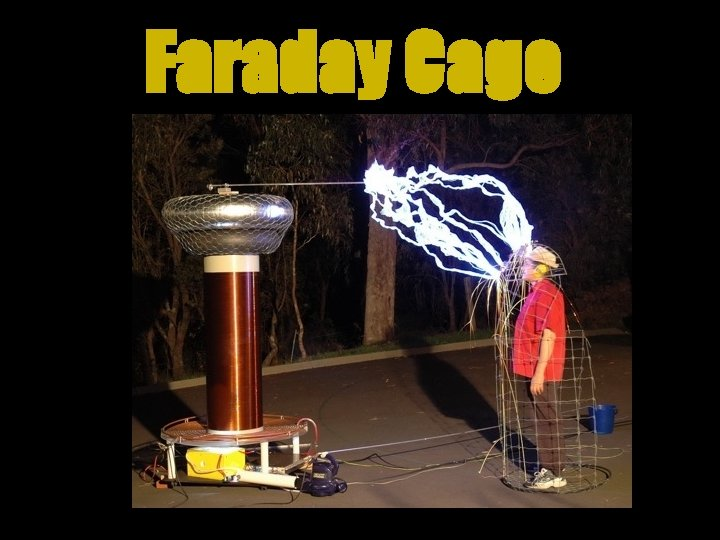# Electrostatics Electric charges at rest static electricity Involves

• Slides: 30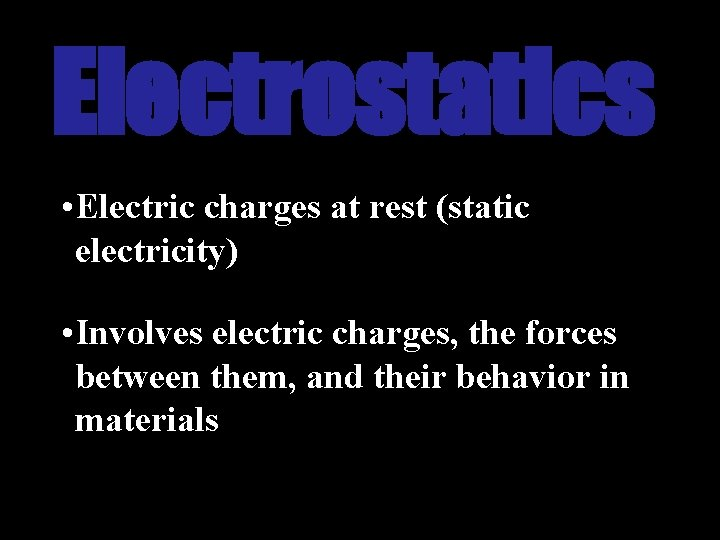Electrostatics • Electric charges at rest (static electricity) • Involves electric charges, the forces between them, and their behavior in materials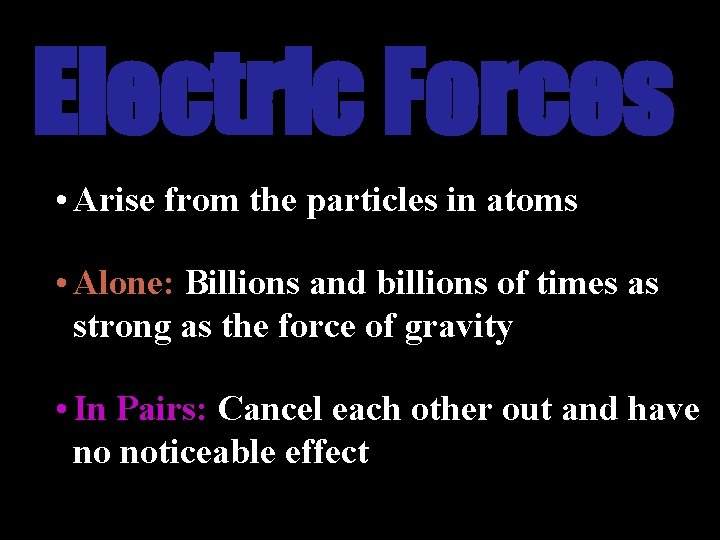Electric Forces • Arise from the particles in atoms • Alone: Billions and billions of times as strong as the force of gravity • In Pairs: Cancel each other out and have no noticeable effect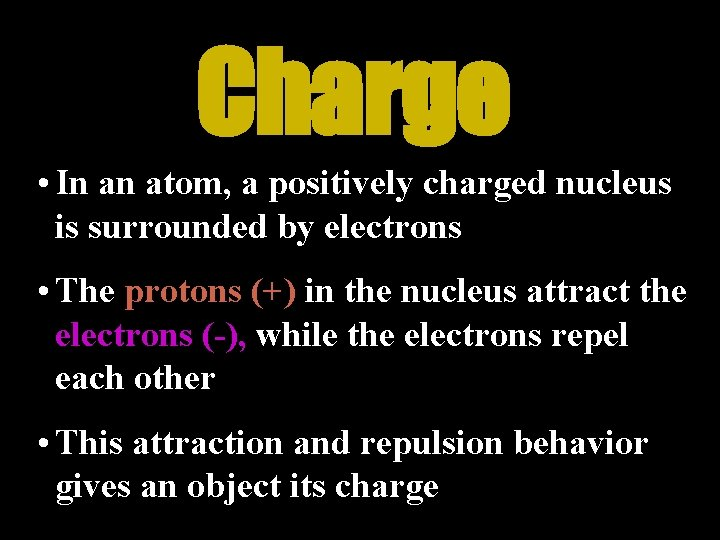Charge • In an atom, a positively charged nucleus is surrounded by electrons • The protons (+) in the nucleus attract the electrons (-), while the electrons repel each other • This attraction and repulsion behavior gives an object its charge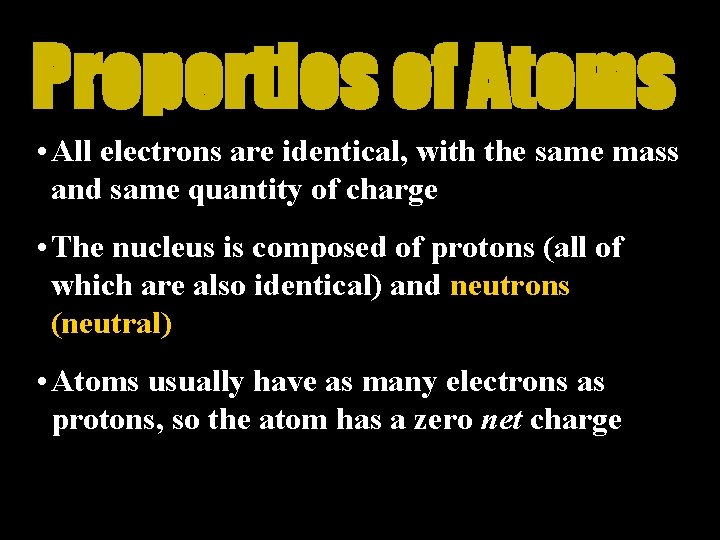Properties of Atoms • All electrons are identical, with the same mass and same quantity of charge • The nucleus is composed of protons (all of which are also identical) and neutrons (neutral) • Atoms usually have as many electrons as protons, so the atom has a zero net chargeThe Fundamental Rule… Like charges repel; opposite charges attract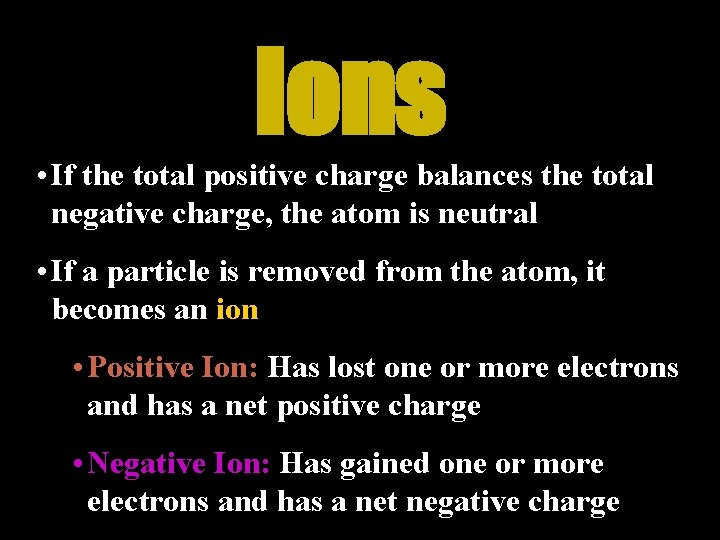Ions • If the total positive charge balances the total negative charge, the atom is neutral • If a particle is removed from the atom, it becomes an ion • Positive Ion: Has lost one or more electrons and has a net positive charge • Negative Ion: Has gained one or more electrons and has a net negative chargeRemoval of Electrons • The inner electrons are bound very tightly to the atom • The outermost electrons of many atoms are bound loosely and can be easily dislodgedConductors and Insulators • Conductor: Materials which allow electric charge to flow freely • Metals are good conductors because their outer electrons are not bound tightly • Insulator: Materials which do not allow electric charge to flow freely (i. e. glass, rubber)Conductors and Insulators • Semiconductor: Materials that can be made to behave as either a conductor or an insulator of electricity • i. e. ) germanium, silicon • Superconductor: Material that has infinite conductivity at low temperatures so that charge flows through it without resistanceConservation of Charge Electrons are never created nor destroyed, but are simply transferred from one material to another • No case of the creation or destruction of net electric charge has ever been found • Electrons are always transferred in whole – they cannot be divided into fractions of electrons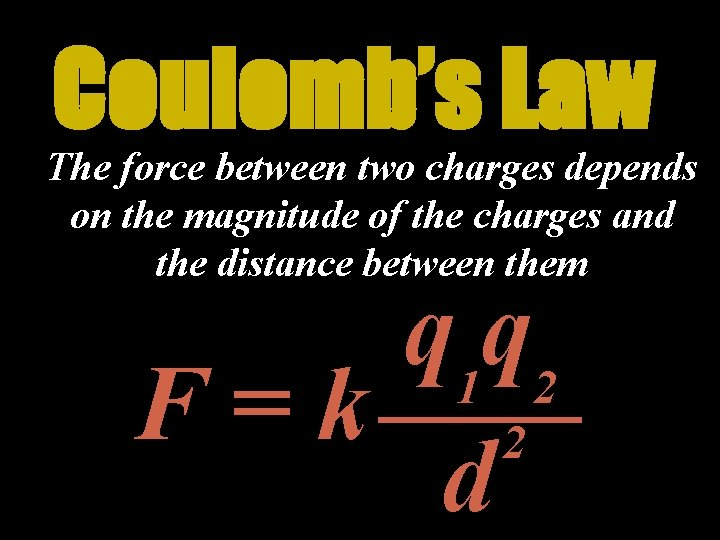Coulomb’s Law The force between two charges depends on the magnitude of the charges and the distance between them q 1 q 2 F=k 2 d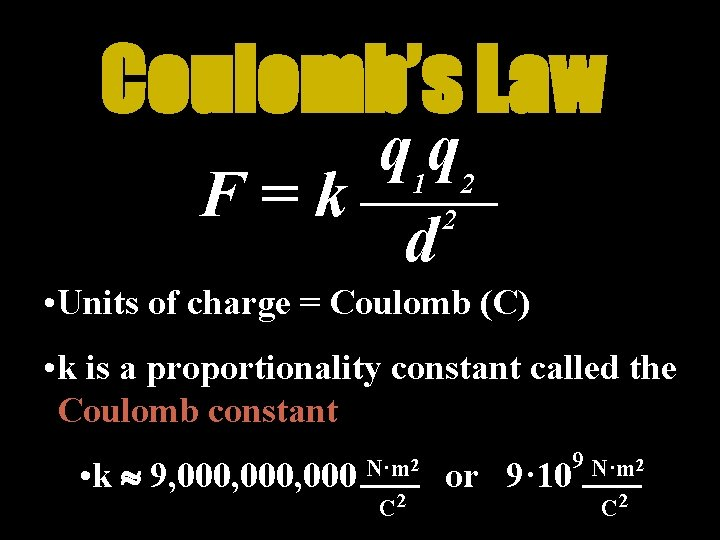Coulomb’s Law q 1 q 2 F=k 2 d • Units of charge = Coulomb (C) • k is a proportionality constant called the Coulomb constant • k 9, 000, 000 N·m 2 C 2 or 9· 10 9 N·m 2 C 2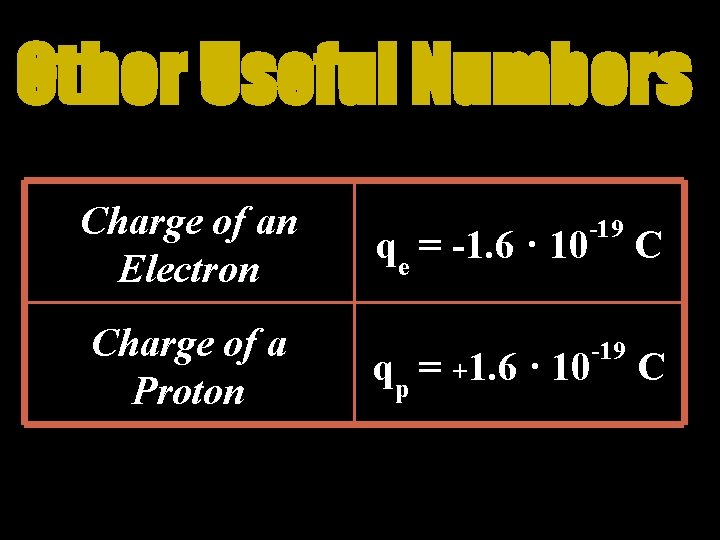Other Useful Numbers Charge of an Electron Charge of a Proton qe = -1. 6 · 10 -19 C qp = +1. 6 · 10Coulomb’s Law The force between two charges depends on the magnitude of the charges and the distance between them q 1 q 2 F=k 2 dSample Problem Two electrons are a meter apart. What is the force between them? What direction is it in?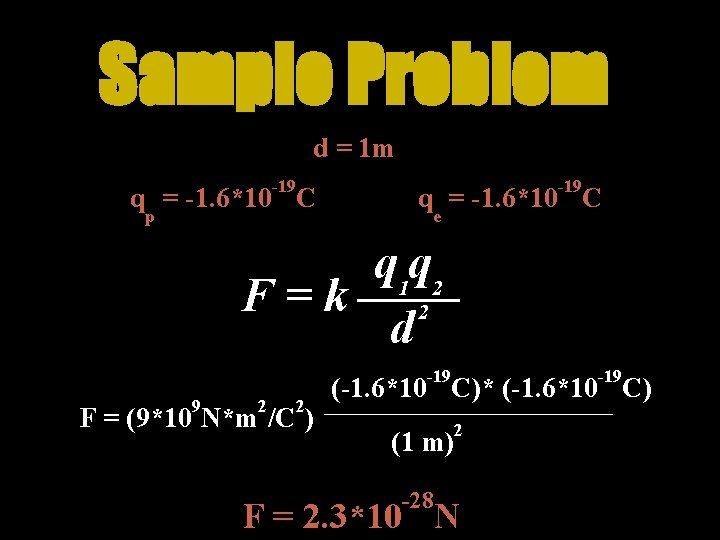Sample Problem d = 1 m -19 q = -1. 6*10 C p -19 q = -1. 6*10 C e q 1 q 2 F=k 2 d -19 9 2 2 F = (9*10 N*m /C ) -19 (-1. 6*10 C)* (-1. 6*10 C) (1 m) -28 2 F = 2. 3*10 N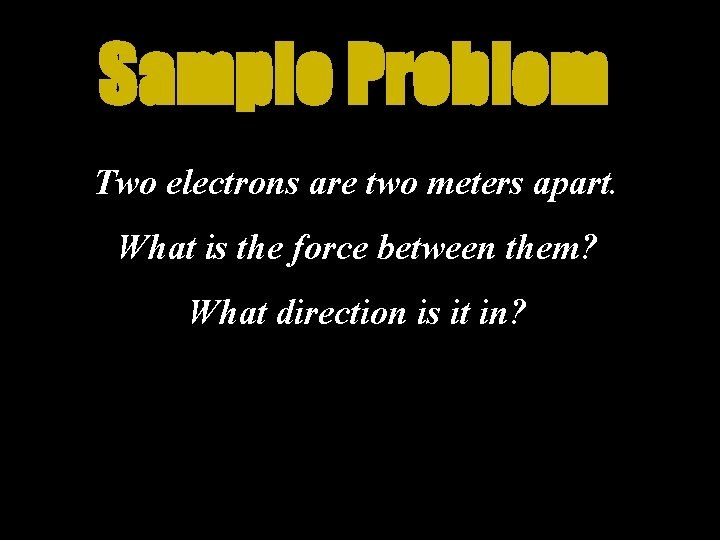Sample Problem Two electrons are two meters apart. What is the force between them? What direction is it in?Coulomb’s Law The force between the two particles falls off as 1/d 2 q 1 q 2 F=k 2 d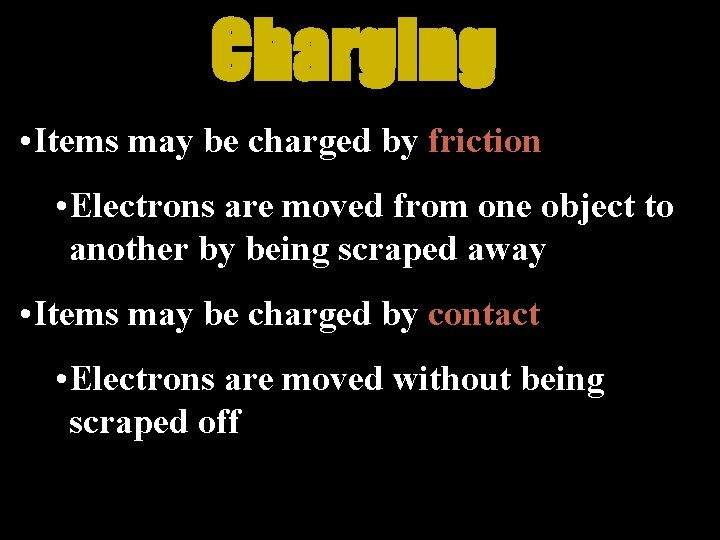Charging • Items may be charged by friction • Electrons are moved from one object to another by being scraped away • Items may be charged by contact • Electrons are moved without being scraped offCharging by Induction: The charging of an object without direct contact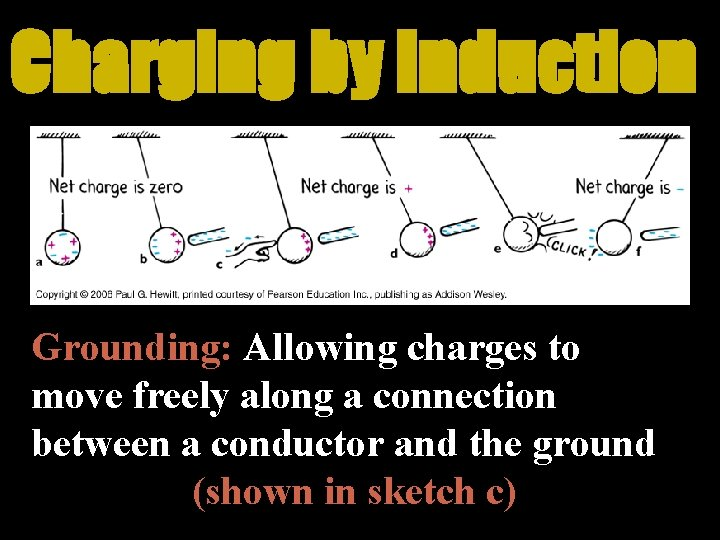Charging by Induction Grounding: Allowing charges to move freely along a connection between a conductor and the ground (shown in sketch c)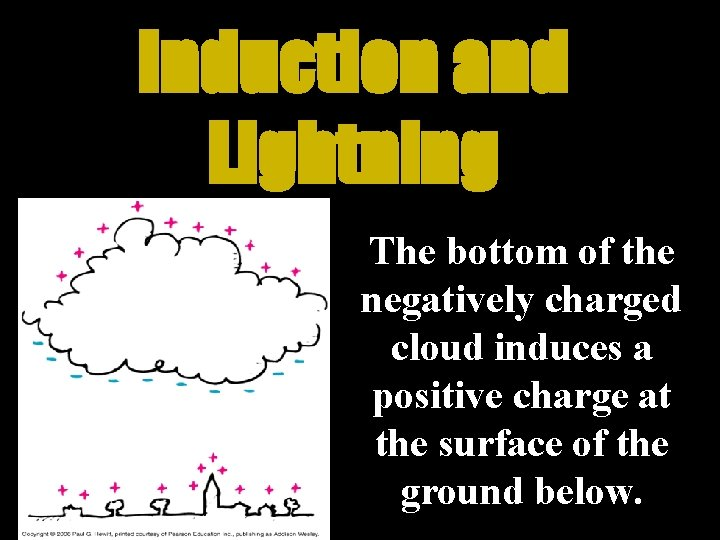Induction and Lightning The bottom of the negatively charged cloud induces a positive charge at the surface of the ground below.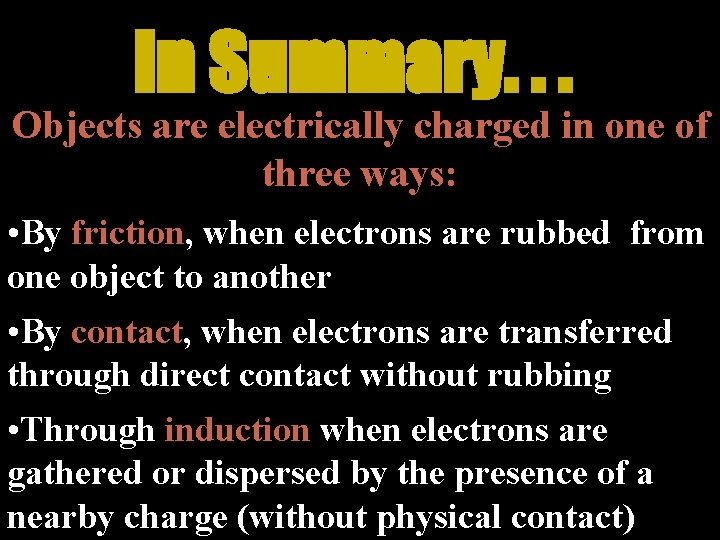In Summary. . . Objects are electrically charged in one of three ways: • By friction, when electrons are rubbed from one object to another • By contact, when electrons are transferred through direct contact without rubbing • Through induction when electrons are gathered or dispersed by the presence of a nearby charge (without physical contact)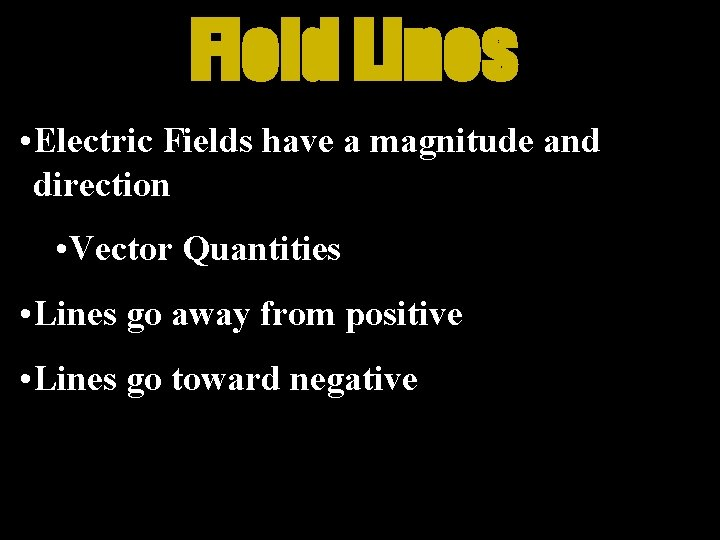Field Lines • Electric Fields have a magnitude and direction • Vector Quantities • Lines go away from positive • Lines go toward negativeDrawing Field Lines • From + to – • Lines start perpendicular to the surface of the charge • Field strength is shown by the density of the field linesElectric Shielding • All charge on a conductor gathers on the outside • If a charge is contained inside a conductor the electric field is zero • If a charge is outside a conductor the inside of the container will not be affected by the outside charge • Example: Faraday Cage